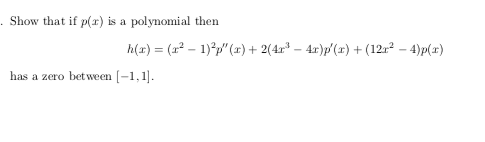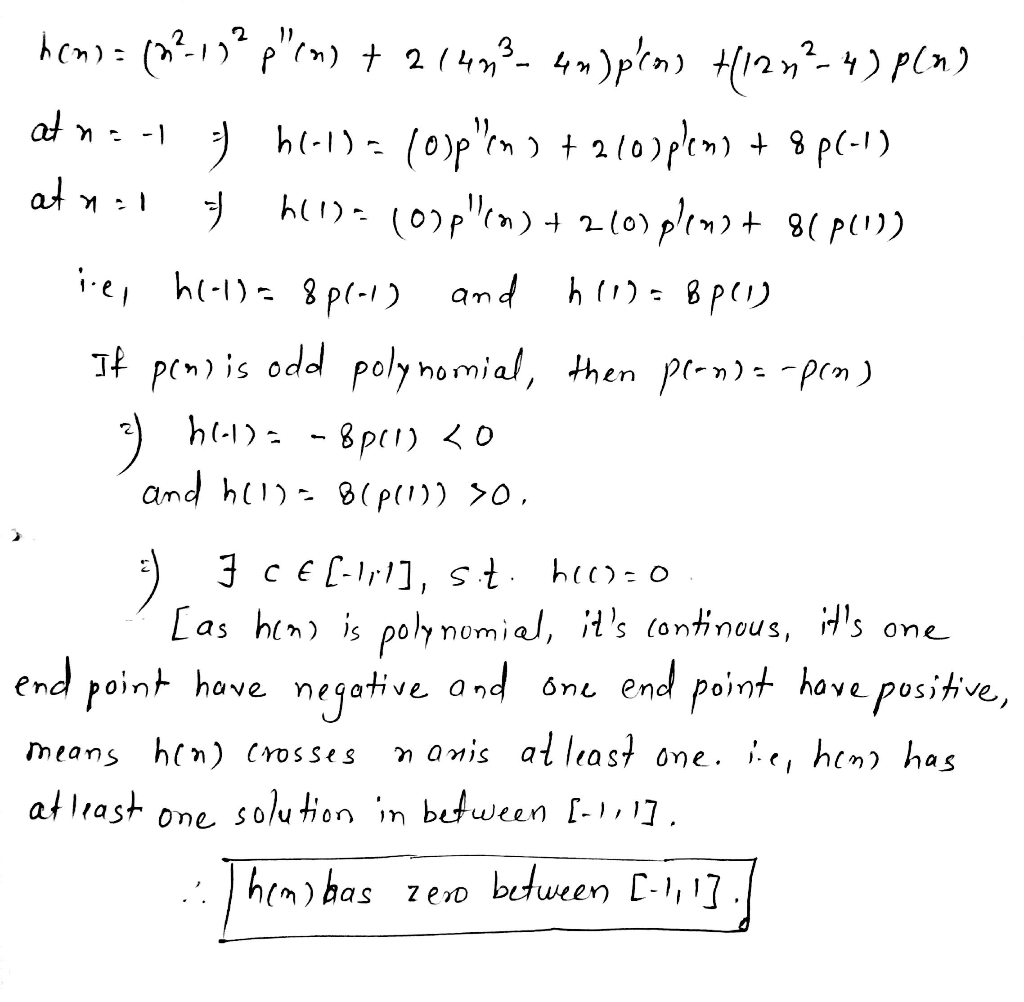# Show that if p(r) is a polynomial then h(x) (2-1)2")2(44)(x)122 -4)px) has a zero bet ween [-1,1Show that if p(r) is a polynomial then h(x) (2-1)2")2(44)(x)122 -4)px) has a zero bet ween [-1,1##### Add Answer of: Show that if p(r) is a polynomial then h(x) (2-1)2")2(44)(x)122 -4)px) has a zero bet ween [-1,1
More Homework Help Questions Additional questions in this topic.

• #### essential diff between b/ween prokarote and eukaryote

Need Online Homework Help?